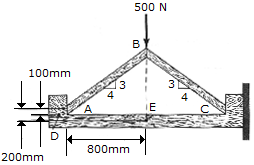# Engineering Mechanics - Internal Forces - Discussion

### Discussion :: Internal Forces - General Questions (Q.No.4)

4.A force of 500 N acts at the top of the two-member frame. If the members are in smooth contact with one another at A, B, and C with no fasteners, determine the shear force developed at a horizontal section through point D of the support. Also, what are the axial force, shear force and moment at point E?

 [A]. VD = 250 N, AE = 250 N, VE = 333 N, ME = 316 N-m [B]. VD = 333 N, AE = 333 N, VE = 250 N, ME = 267 N-m [C]. VD = 333 N, AE = 333 N, VE = 250 N, ME = 200 N-m [D]. VD = 250 N, AE = 250 N, VE = 333 N, ME = 267 N-m

Explanation:

No answer description available for this question.

 Sheela said: (Mar 10, 2015) Please explain clear.